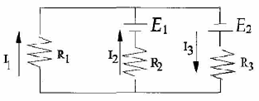# Problem: The following equation is true for the right loop of the circuit. a) E2 - I3R3 - I2R2 - E1 = 0 b) -E2 - I3R3 - I2R2 - E1 = 0 c) E2 - I3R3 - I2R2 + E1 = 0 d) E2 + I3R3 + I2R2 - E1 = 0 e) None of the above

###### Problem Details

The following equation is true for the right loop of the circuit.

a) E2 - I3R3 - I2R2 - E1 = 0

b) -E2 - I3R3 - I2R2 - E1 = 0

c) E2 - I3R3 - I2R2 + E1 = 0

d) E2 + I3R3 + I2R2 - E1 = 0

e) None of the above